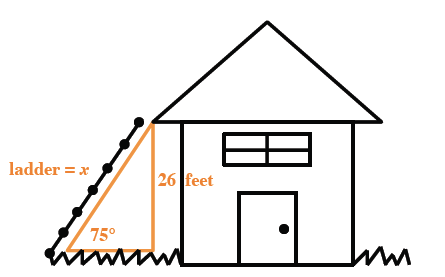### Home > CCG > Chapter 5 > Lesson 5.1.2 > Problem5-18

5-18.

While shopping at his local home improvement store, Chen noticed that the directions for an extension ladder state, “This ladder is most stable when used at a $\mathit{75º}$ angle with the ground.” He wants to buy a ladder to paint a two-story house that is $26$ feet high. How long does his ladder need to be? Draw a diagram and set up an equation for this situation. Show all work.

Draw a diagram comparable to the one below.Refer to the Math Notes box in Lesson 5.1.2 if you need help deciding which trigonometric ratio to use when solving for $x$.

$\text{length of ladder}=x$